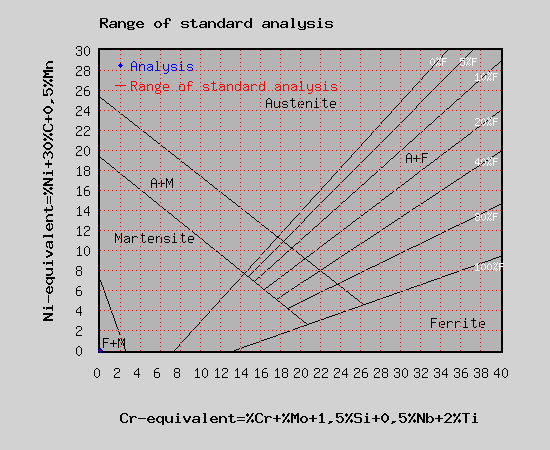# Schaeffler diagram for standard analysis

The Schaeffler diagram provides information on the welding properties of the various types of microstructure, as a function of what alloying elements they contain. Chromium equivalent is calculated using the weight percentage of ferrite stabilising elements and Nickel equivalent is calculated using the weight percentage of austenite stabilising elements. By entering the Ni-equivalent over the Cr-equivalent for stainless steel into a diagram according to Schaeffler one is able to find the content of marteniste, austenite and ferrite in the resulting microstructure.

Calculation

After entering the range of the standard analysis and the actual analysis the Ni-equivalent and the Cr-equivalent are calculated and shown in a diagram.

The sample data can be overridden and the red backgrounded fields show the results.

 Composition Analysis Minimum Maximum Carbon C [%] Silicon Si [%] Manganese Mn [%] Chromium Cr [%] Molybdenum Mo [%] Nickel Ni [%] Niobium Nb [%] Titanium Ti [%] Chromium equivalent  [%] Nickel equivalent  [%]